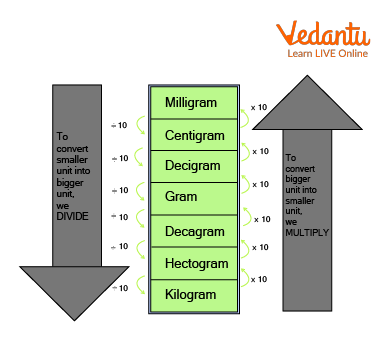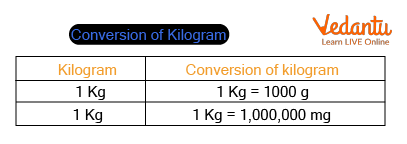Courses
Courses for Kids
Free study material
Free LIVE classes
More

# Kilogram Conversions: How to Convert Kg into GramLIVE
Join Vedantu’s FREE Mastercalss

## How Many Grams in a Kilo?

The term “Measurement” describes the quantity of any object either in a solid or liquid state.

It helps to know the unit of every shape. There are basic units to measure the weight, length, and capacity of different shapes.

Measures of Mass: The quantity of matter or substance that makes up a thing is known as its mass. Mass is the measure of matter in an object. A unit of measurement is kilograms, abbreviated as kg.Which is Heavier?

Can you tell us which one is heavier in the above image? Whose weight would be in Kg?

Yes, which one is heavy is depicted by the size of small and large but to know how much, we need to use a tool like a weighing machine to know the accurate weight.

Mass v/s Weight: It's crucial to keep in mind that mass differs from the weight. While weight varies with changes in gravity, mass never changes.

Weighing is how we determine the mass, yet weight and mass are not the same.

The most typical measurements are as follows:

• Grams

• Kilograms

• Tonnes

Tonnes are the largest, while Grams are the smallest.

Here, the kilogram conversion is explained in detail. Few solved examples are shown below on how to convert kg into grams.

## Grams

An average paper clip weighs one gram.Paperclip

Let’s understand this through an activity.

Activity: One little paperclip should be held in your hand. Does that have much weight? No! Grams are quite light. This explains why items are frequently measured in hundreds of grams.

Since the abbreviation "g" for grams is frequently used, "300 g" stands for "300 grams."

The average loaf of bread weighs 700 g. (for a nice-sized loaf).

## Kilograms

• A kilogram is a unit used to measure mass.

• Kilograms are excellent for weighing objects that people can lift, yet occasionally very powerful individuals are required.

• 1,000 grams make up 1 kilogram.

For example, an average dictionary weighs roughly one kilogram.Dictionary

Another example is 1 kilogram of gold. The weight of this gold bar is similarly one kilogram.Gold

Since kilograms are sometimes written as kg ("k" for "kilo" and "g" for "gramme"), "10 kg" refers to ten kilograms.Weighing Machine

Our weight is measured on scales in kilograms. An adult typically weighs 70 kg.

But we must use the tonne when referring to extremely hefty items.

## Kilogram Conversion

There are seven basic units used in kilogram conversion.

• Milligrams

• Centigrams

• Decigrams

• Gram

• Decagrams

• Hectograms

• Kilograms

This is present in decreasing order means, a milligram is the smallest and a kilogram is the highest. To convert a smaller unit into a bigger unit we divide it by 10 and to convert a bigger into a smaller we multiply it by 10.Conversion

## Grams to Kilograms

The International System of Units also defines a gram as a unit of mass (SI). It weighs 0.001 kilograms. It is used to measure light things like pens and candy bars and is represented by the sign "g".

As a result, 1 kilogram (Kg) equals 1000 grams is the relationship between kilogram and grams (g).

Grams to Kilogram = 1g = 0.001 kg

Kilogram to Gram = 1 kg = 1000 g

## Kilogram to Milligrams

As 1 gram (g) is equal to 1000 milligrams (mg), hence the relation between kilogram and milligrams is given as, 1 kilogram (kg) = 1000000 milligrams (mg).Conversion of Kilogram

Below are the few solved examples and practice problems on how to convert kg into gram.

## Solved Example

Q1. Convert the following kilogram into grams.

(a) $2 \mathrm{~kg}$ into $\mathrm{g}$

Ans: $1 \mathrm{~kg}=1000 \mathrm{~g}$

$2 \mathrm{~kg}=2 \times 1000 \mathrm{~g}$

$=2000 \mathrm{~g}$

(b) $5 \mathrm{~g}$ into $\mathrm{kg}$

Ans: $1 \mathrm{~g}=\dfrac{1}{1000} \mathrm{~kg}$

$5 \mathrm{~g}=(\dfrac{5}{1000}) \mathrm{kg}$

$=0.005 \mathrm{~kg}$

(c) $1 \mathrm{~kg} 506 \mathrm{~g}$ into $\mathrm{g}$

Ans: $1 \mathrm{~kg}=1000 \mathrm{~g}$

$1 \mathrm{~kg} 506 \mathrm{~g}=1 \times 1000 \mathrm{~g}+506 \mathrm{~g}$

$=1000 \mathrm{~g}+506 \mathrm{~g}$

$=1506 \mathrm{~g}$

(d) $5 \mathrm{~kg} 124 \mathrm{~g}$ into $\mathrm{g}$

Ans: $1 \mathrm{~kg}=1000 \mathrm{~g}$

$5 \mathrm{~kg} 124 \mathrm{~g}=(5 \times 1000) \mathrm{g}+124 \mathrm{~g}$

$=5000 \mathrm{~g}+124 \mathrm{~g}$

$=5124 \mathrm{~g}$

(e) $15 \mathrm{~g}$ into $\mathrm{kg}$

Ans: $1 \mathrm{~kg}=1000 \mathrm{~g}$

$15 \mathrm{~g}=(15 \times 1000) \mathrm{g}$

$=15000 \mathrm{~g}$

## Practice Problems

1. Convert the following:

(a) 5g into kg

(b) 5kg 64g into kg

(c) 6kg into g

Ans:

(a) 0.005kg

(b) 5.064kg

(c) 0.006g

## Summary

The kilogram is employed to weigh things with a medium to high density. Body weight, vegetables, and fruits are all measured in kilograms, which are represented by the sign "kg," where "k" stands for kilo and "g" for gram. There are so many different-sized and-weighted items all around us. The same mass is being measured in different units. There are different units of measurement of mass. Like Kilogram, milligram, centigram, etc.

Last updated date: 29th Sep 2023
Total views: 126.3k
Views today: 1.26k

## FAQs on Kilogram Conversions: How to Convert Kg into Gram

1. What are kilograms and grams?

The primary mass units in the SI and CGS systems are the kilogram and the gram, respectively. The metric system uses kilograms as the unit of mass. The fundamental mass unit used to convert between grams and kilograms is the gram.

Both of these units of measurement allow us to determine the mass of an object. Because grams are smaller than kilograms, they are used to measure smaller objects. much smaller! One kilogram is made up of 1,000 grams!

2. Are mass and weight the same?

An object's mass is an important measure of how much matter it contains. Weight is a measurement of an object's gravitational pull. It is influenced by the object's location in addition to its mass. As a result, weight is a measurement of force.

Weight and mass are not the same. Weight is a measurement of an object's gravitational pull. An object's weight can vary depending on where it is, but its mass never changes.

For instance, even if you weigh 100 pounds on Earth, you would have no weight there.